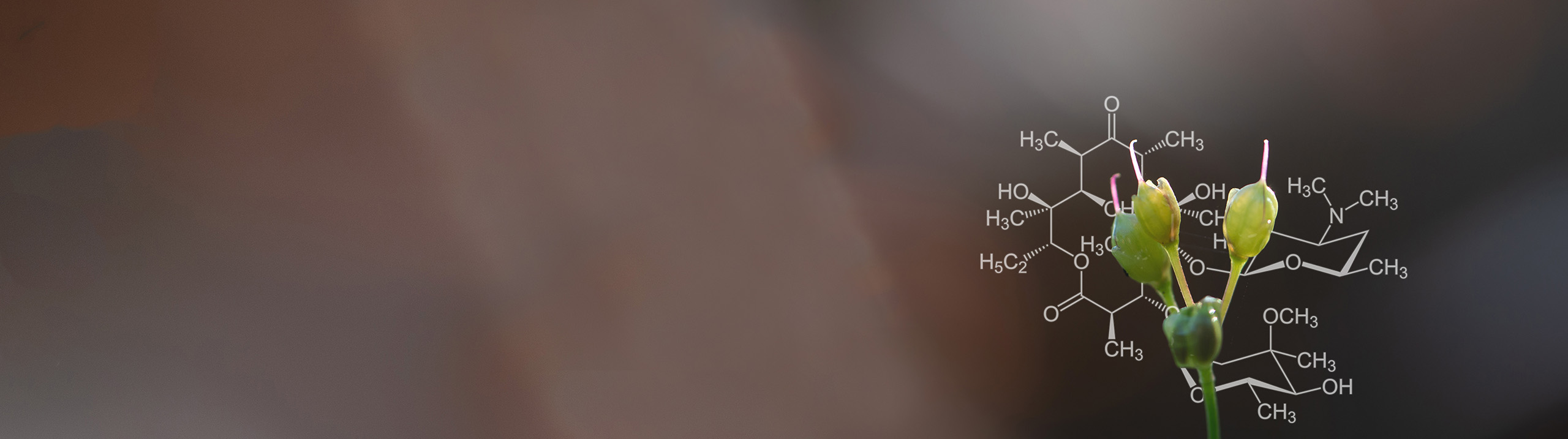Start typing, then use the up and down aroows to select an option from the list.# Biochemistry

Learn the toughest concepts covered in Biochemistry with step-by-step video tutorials and practice problems by world-class tutors

11. Biological Membranes and Transport

# Thermodynamics of Membrane Diffusion: Charged Ion

1

### concept

Thermodynamics of Membrane Diffusion: Charged Ion6m
Play a video:
0
2

### example

Thermodynamics of Membrane Diffusion: Charged Ion Example 115m
Play a video:
0
3
Problem

Calculate the free energy change (ΔG transport) for the movement of Na + into a cell when its concentration outside is 150 mM and its cytosolic concentration is 10 mM. Assume that T = 20°C and ΔΨ = –50 mV (inside negative).

4
Problem

Calculate the ΔGtransport required to move 1 mole of Na + ions from inside the cell ([Na+] inside = 5 mM) to the outside of the cell ([Na +] outside = 150 mM) when ΔΨ = –70 mV (inside negative) & the temperature is 37°C.

5
Problem

Calculate the ΔGtransport when Ca2+ ions move from the endoplasmic reticulum ([Ca2+] = 1 mM) to the cytoplasm ([Ca2+] = 0.1 μM). Assume that ΔΨ = 0 and T = 25°C.